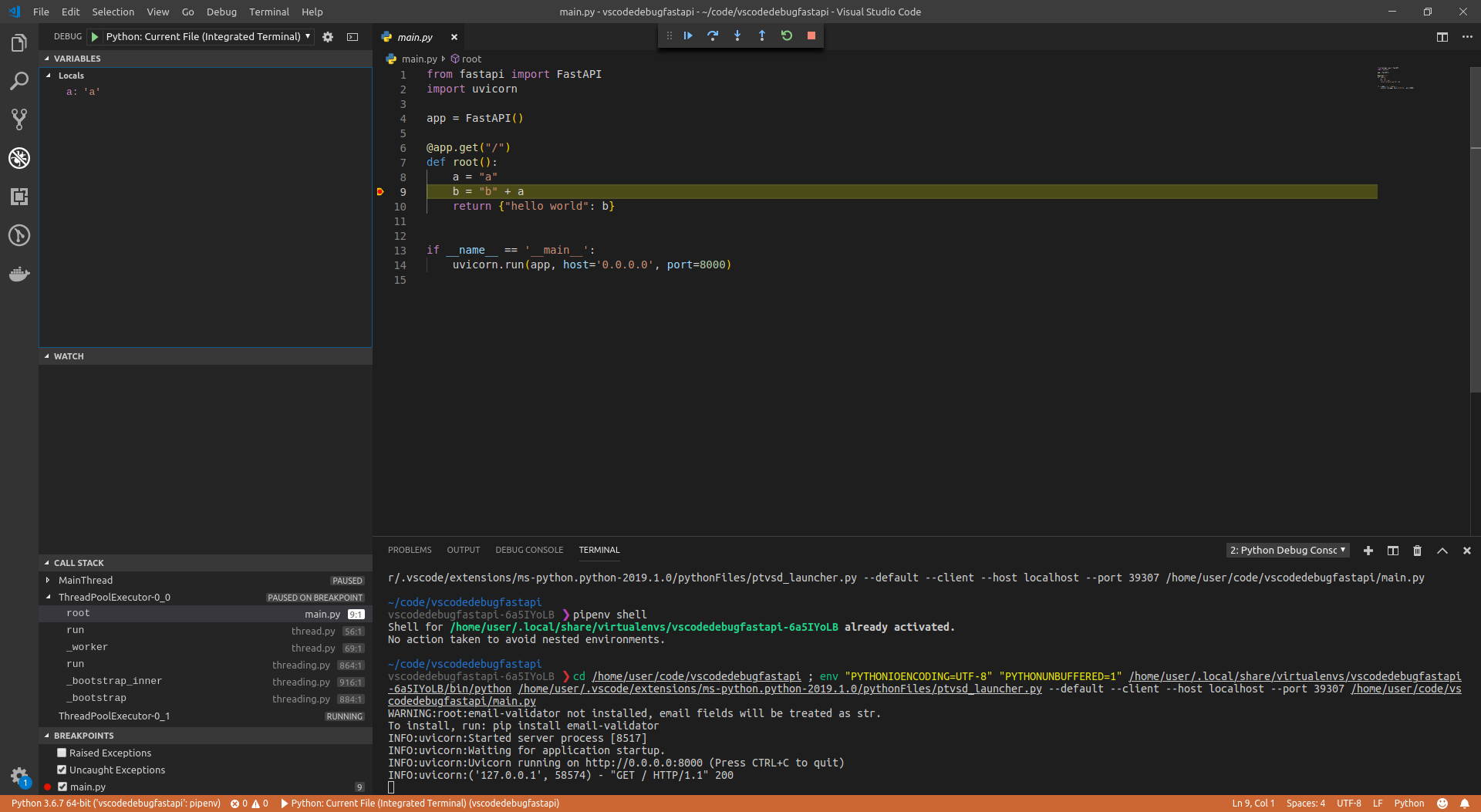# FastApi教程|调试

## 呼叫 ``` uvicorn ```

``````import uvicorn
from fastapi import FastAPI

app = FastAPI()

@app.get("/")
def root():
a = "a"
b = "b" + a
return {"hello world": b}

if __name__ == "__main__":
uvicorn.run(app, host="0.0.0.0", port=8000)
``````

### 关于 ``` __name__ == "__main__" ```

``````python myapp.py
``````

``````from myapp import app
``````

#### 更多细节

``````python myapp.py
``````

``````    uvicorn.run(app, host="0.0.0.0", port=8000)
``````

``````from myapp import app

# Some more code
``````

``````    uvicorn.run(app, host="0.0.0.0", port=8000)
``````

## 使用调试器运行代码

• 转到“调试”面板。
• “添加配置...”。
• 选择“ Python”
• 使用选项“ ``` Python: Current File (Integrated Terminal) ``` ” 运行调试器 。Python Free

QQ：417803890